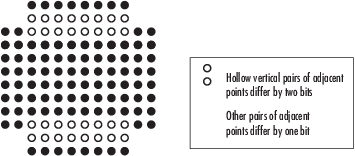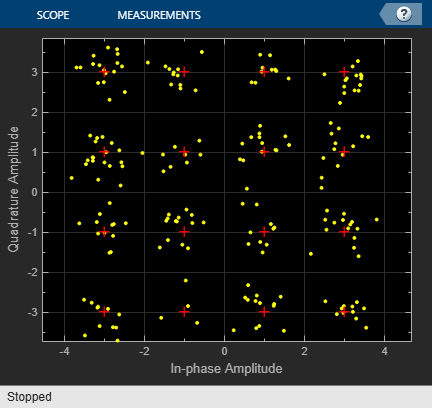# Rectangular QAM Modulator Baseband

Modulate using rectangular quadrature amplitude modulation

## Library

AM, in Digital Baseband sublibrary of Modulation

•## Description

The Rectangular QAM Modulator Baseband block modulates using M-ary quadrature amplitude modulation with a constellation on a rectangular lattice. The output is a baseband representation of the modulated signal. This block accepts a scalar or column vector input signal. For information about the data types each block port supports, see Supported Data Types.

Note

All values of power assume a nominal impedance of 1 ohm.

### Integer-Valued Signals and Binary-Valued Signals

When you set the Input type parameter to `Integer`, the block accepts integer values between `0` and M-`1`. M represents the M-ary number block parameter.

When you set the Input type parameter to `Bit`, the block accepts binary-valued inputs that represent integers. The block collects binary-valued signals into groups of K = log2(M) bits

where

K represents the number of bits per symbol.

The input vector length must be an integer multiple of K. In this configuration, the block accepts a group of K bits and maps that group onto a symbol at the block output. The block outputs one modulated symbol for each group of K bits.

The Constellation ordering parameter indicates how the block assigns binary words to points of the signal constellation. Such assignments apply independently to the in-phase and quadrature components of the input:

• If Constellation ordering is set to `Binary`, the block uses a natural binary-coded constellation.

• If Constellation ordering is set to `Gray` and K is even, the block uses a Gray-coded constellation.

• If Constellation ordering is set to `Gray` and K is odd, the block codes the constellation so that pairs of nearest points differ in one or two bits. The constellation is cross-shaped, and the schematic below indicates which pairs of points differ in two bits. The schematic uses M = 128, but suggests the general case.For details about the Gray coding, see the reference page for the M-PSK Modulator Baseband block and the paper listed in References. Because the in-phase and quadrature components are assigned independently, the Gray and binary orderings coincide when M = 4.

### Constellation Size and Scaling

The signal constellation has M points, where M is the M-ary number parameter. M must have the form 2K for some positive integer K. The block scales the signal constellation based on how you set the Normalization method parameter. The following table lists the possible scaling conditions.

Value of Normalization Method ParameterScaling Condition
`Min. distance between symbols` The nearest pair of points in the constellation is separated by the value of the Minimum distance parameter
`Average Power` The average power of the symbols in the constellation is the Average power parameter
`Peak Power` The maximum power of the symbols in the constellation is the Peak power parameter

## Parameters

M-ary number

The number of points in the signal constellation. It must have the form 2K for some positive integer K.

Input type

Indicates whether the input consists of integers or groups of bits.

Constellation ordering

Determines how the block maps each symbol to a group of output bits or integer.

Selecting `User-defined` displays the field Constellation mapping, which allows for user-specified mapping.

Constellation mapping

This parameter is a row or column vector of size M and must have unique integer values in the range [0, M-1]. The values must be of data type `double`.

The first element of this vector corresponds to the top-leftmost point of the constellation, with subsequent elements running down column-wise, from left to right. The last element corresponds to the bottom-rightmost point.

This field appears when `User-defined` is selected in the drop-down list Constellation ordering.

Normalization method

Determines how the block scales the signal constellation. Choices are `Min. distance between symbols`, `Average Power`, and ```Peak Power```.

Minimum distance

The distance between two nearest constellation points. This field appears only when Normalization method is set to `Min. distance between symbols`.

Average power, referenced to 1 ohm (watts)

The average power of the symbols in the constellation, referenced to 1 ohm. This field appears only when Normalization method is set to `Average Power`.

Peak power, referenced to 1 ohm (watts)

The maximum power of the symbols in the constellation, referenced to 1 ohm. This field appears only when Normalization method is set to `Peak Power`.

The rotation of the signal constellation, in radians.

Output data type

The output data type can be set to `double`, `single`, `Fixed-point`, `User-defined`, or ```Inherit via back propagation```.

Setting this parameter to `Fixed-point` or `User-defined` enables fields in which you can further specify details. Setting this parameter to ```Inherit via back propagation```, sets the output data type and scaling to match the following block.

Output word length

Specify the word length, in bits, of the fixed-point output data type. This parameter is only visible when you select `Fixed-point` for the Output data type parameter.

User-defined data type

Specify any signed built-in or signed fixed-point data type. You can specify fixed-point data types using the `sfix` (Simulink), `sint` (Simulink), `sfrac` (Simulink), and `fixdt` (Simulink) functions from Fixed-Point Designer™ software. This parameter is only visible when you select `User-defined` for the Output data type parameter.

Set output fraction length to

Specify the scaling of the fixed-point output by either of the following methods:

• Choose `Best precision` to have the output scaling automatically set such that the output signal has the best possible precision.

• Choose `User-defined` to specify the output scaling in the Output fraction length parameter.

This parameter is only visible when you select `Fixed-point` for the Output data type parameter or when you select `User-defined` and the specified output data type is a fixed-point data type.

Output fraction length

For fixed-point output data types, specify the number of fractional bits, or bits to the right of the binary point. This parameter is only visible when you select `Fixed-point` or `User-defined` for the Output data type parameter and `User-defined` for the Set output fraction length to parameter.

## Examples

expand all

Use the Open model button to open the `doc_qam_mod` model. The model generates a QAM signal, applies white noise, and displays the resulting constellation diagram.Run the model.Change the Eb/No of the AWGN Channel block from 20 dB to 10 dB. Observe the increase in the noise.## Supported Data Types

PortSupported Data Types

Input

• Double-precision floating point

• Single-precision floating point

• Boolean when Input type is `Bit`

• 8-, 16-, 32-bit signed integers

• 8-, 16-, 32-bit unsigned integers

• $ufix\left({\mathrm{log}}_{2}M\right)$ when Input type is `Integer`

Output

• Double-precision floating point

• Single-precision floating point

• Signed fixed-point

## Pair Block

Rectangular QAM Demodulator Baseband

## References

 Smith, Joel G., “Odd-Bit Quadrature Amplitude-Shift Keying,” IEEE Transactions on Communications, Vol. COM-23, March 1975, 385–389.

expand all

## Extended Capabilities

### Functions

Introduced before R2006a